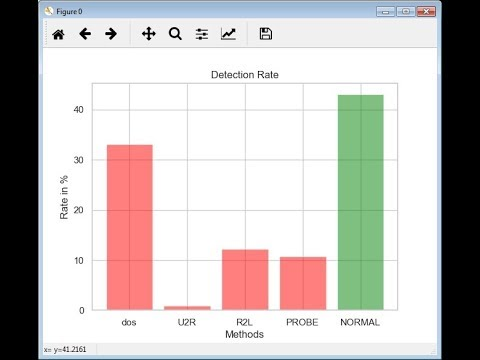# Multi-class intrusion detection using machine learning algorithms

Implementation Details:

We have used KDD dataset with multiple class intrusion namely DDOS, U2R, R2L, Probe, Normal, Unknown

We have pre-processed dataset by using the following numbers instead of attack type (class value)
dos = 
u2r = 
r2l = 
probe = 
normal = 
unknown = 

We applied machine learning algorithm namely, Logistic regression, Decision Tree and Random Forest.
Out of which Logistic regression outperforms interms of accuracy

Python Demo